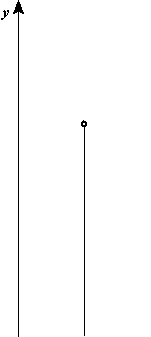# 2.5: Free-Falling Objects

•• Boundless
• Boundless
$$\newcommand{\vecs}{\overset { \rightharpoonup} {\mathbf{#1}} }$$ $$\newcommand{\vecd}{\overset{-\!-\!\rightharpoonup}{\vphantom{a}\smash {#1}}}$$$$\newcommand{\id}{\mathrm{id}}$$ $$\newcommand{\Span}{\mathrm{span}}$$ $$\newcommand{\kernel}{\mathrm{null}\,}$$ $$\newcommand{\range}{\mathrm{range}\,}$$ $$\newcommand{\RealPart}{\mathrm{Re}}$$ $$\newcommand{\ImaginaryPart}{\mathrm{Im}}$$ $$\newcommand{\Argument}{\mathrm{Arg}}$$ $$\newcommand{\norm}{\| #1 \|}$$ $$\newcommand{\inner}{\langle #1, #2 \rangle}$$ $$\newcommand{\Span}{\mathrm{span}}$$ $$\newcommand{\id}{\mathrm{id}}$$ $$\newcommand{\Span}{\mathrm{span}}$$ $$\newcommand{\kernel}{\mathrm{null}\,}$$ $$\newcommand{\range}{\mathrm{range}\,}$$ $$\newcommand{\RealPart}{\mathrm{Re}}$$ $$\newcommand{\ImaginaryPart}{\mathrm{Im}}$$ $$\newcommand{\Argument}{\mathrm{Arg}}$$ $$\newcommand{\norm}{\| #1 \|}$$ $$\newcommand{\inner}{\langle #1, #2 \rangle}$$ $$\newcommand{\Span}{\mathrm{span}}$$$$\newcommand{\AA}{\unicode[.8,0]{x212B}}$$

learning objectives

• Solve basic problems concerning free fall and distinguish it from other kinds of motion

The motion of falling objects is the simplest and most common example of motion with changing velocity. If a coin and a piece of paper are simultaneously dropped side by side, the paper takes much longer to hit the ground. However, if you crumple the paper into a compact ball and drop the items again, it will look like both the coin and the paper hit the floor simultaneously. This is because the amount of force acting on an object is a function of not only its mass, but also area. Free fall is the motion of a body where its weight is the only force acting on an object.Free Fall: This clip shows an object in free fall.

Galileo also observed this phenomena and realized that it disagreed with the Aristotle principle that heavier items fall more quickly. Galileo then hypothesized that there is an upward force exerted by air in addition to the downward force of gravity. If air resistance and friction are negligible, then in a given location (because gravity changes with location), all objects fall toward the center of Earth with the same constant acceleration, independent of their mass, that constant acceleration is gravity. Air resistance opposes the motion of an object through the air, while friction opposes motion between objects and the medium through which they are traveling. The acceleration of free-falling objects is referred to as the acceleration due to gravity gg. As we said earlier, gravity varies depending on location and altitude on Earth (or any other planet), but the average acceleration due to gravity on Earth is 9.8 $$\mathrm{\frac{m}{s^2}}$$. This value is also often expressed as a negative acceleration in mathematical calculations due to the downward direction of gravity.

The best way to see the basic features of motion involving gravity is to start by considering straight up and down motion with no air resistance or friction. This means that if the object is dropped, we know the initial velocity is zero. Once the object is in motion, the object is in free-fall. Under these circumstances, the motion is one-dimensional and has constant acceleration, gg. The kinematic equations for objects experiencing free fall are:

\begin{align} \mathrm{v} & \mathrm{=v_0−gt} \\ \mathrm{y} & \mathrm{=y_0+v_0t−\frac{1}{2}gt^2} \\ \mathrm{v^2} & \mathrm{=v^2_0−2g(y−y_0),} \end{align}

where $$\mathrm{v=velocity, g=gravity, t=time,}$$ and $$\mathrm{y=vertical \; displacement}$$.

Video $$\PageIndex{1}$$: Free Fall Motion - Describes how to calculate the time for an object to fall if given the height and the height that an object fell if given the time to fall.

Example $$\PageIndex{1}$$:

Some examples of objects that are in free fall include:

• A spacecraft in continuous orbit. The free fall would end once the propulsion devices turned on.
• An stone dropped down an empty well.
• An object, in projectile motion, on its descent.

## Key Points

• The acceleration of free-falling objects is called the acceleration due to gravity, since objects are pulled towards the center of the earth.
• The acceleration due to gravity is constant on the surface of the Earth and has the value of 9.80 $$\mathrm{\frac{m}{s^2}}$$ .

## Glossary

Acceleration

The amount by which a speed or velocity changes within a certain period of time (and so a scalar quantity or a vector quantity).